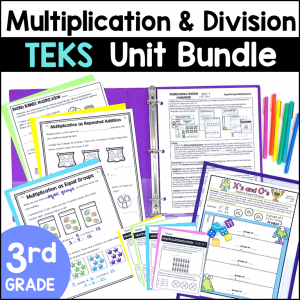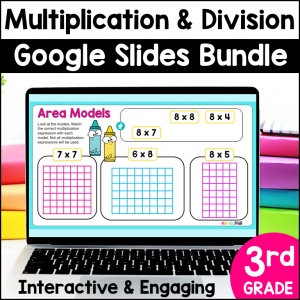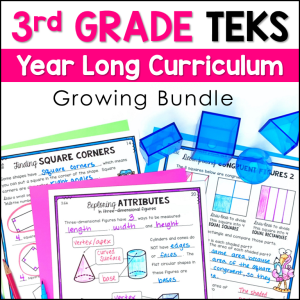Save 10% on your first order with code EXTRA10

# Making Equal Groups Multiplication – Interactive Google Slides

\$0.00\$29.99\$13.49\$151.25
SKU: 8541545 Category:

## Description

Your students will love making equal groups to learn about multiplication with this digital resource. You can use this resource to introduce multiplication while making equal groups during your lesson, or assign it to students to practice making equal groups at a math station. This No Prep activity is perfect for 3rd grade students. Your students will have so much fun moving the interactive pieces to model problems.

Video instructions for students are embedded in the activity, making it easy for you to assign as a no prep math station, for seatwork or homework.

This resource can be easily assigned in Google Classroom or any other platform compatible with Google Slides. Homeschool parents can use this resource by simply opening it in Google Slides. All you need to access Google Slides is a free Gmail account.

What’s Included in this resource?

• Video Instructions (Student devices must be able to access You-Tube)
• 10 student practice slides (10 problems)
• Key

Topics include:

• Making Equal Groups to Model Equations
• Creating Multiplication Equations Based on Models

Ideas on how to use this resource:

• Use it as a whole class teaching tool by projecting the slides and using the movable, interactive pieces to teach your lesson
• Use this resource as a no prep math station
• Use it as an early finisher activity
• Use this resource for small group reteach or tutoring
• Assign this resource as a lesson for student practice as independent work
• Share the digital key for parents and students to check work at home

Standards Alignment:

TEKS

3.4D Determine the total number of objects when equally-sized groups of objects are combined or arranged in arrays up to 10 by 10

3.4E Represent multiplication facts by using a variety of approaches such as repeated addition, equal-sized groups, arrays, area models, equal jumps on a number line, and skip counting

Common Core

3.OA.A.1

Interpret products of whole numbers, e.g., interpret 5 × 7 as the total number of objects in 5 groups of 7 objects each. For example, describe a context in which a total number of objects can be expressed as 5 × 7.